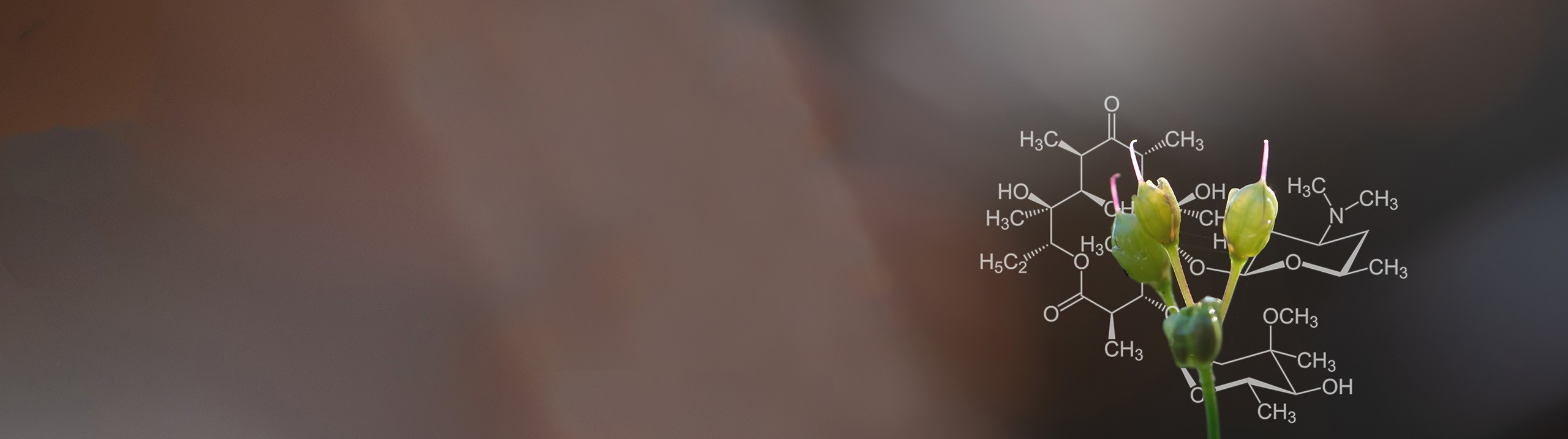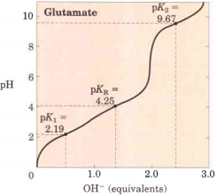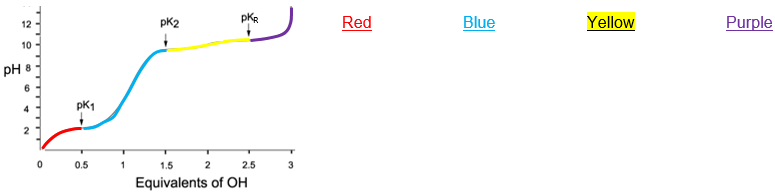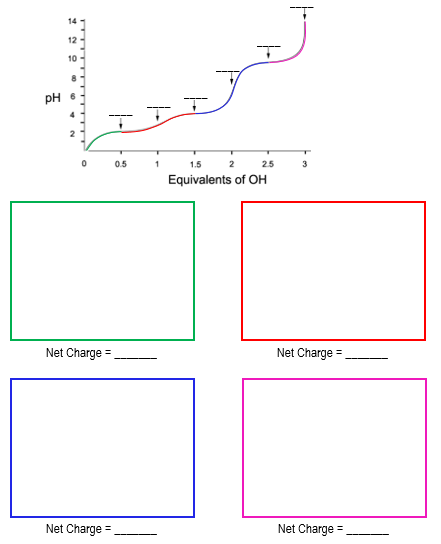Start typing, then use the up and down arrows to select an option from the list.# Biochemistry

Learn the toughest concepts covered in Biochemistry with step-by-step video tutorials and practice problems by world-class tutors

3. Amino Acids

# Titrations of Amino Acids with Ionizable R-Groups

1

#### concept

Titrations Of Amino Acids With Ionizable R-Groups9m
Play a video:
2

#### concept

Titrations Of Amino Acids With Ionizable R-Groups7m
Play a video:
So in our last lesson video, we reviewed the Thai trey shins of amino acids with Ayan Izabal are groups, and in this video we're going to talk about how to draw the predominant structures of amino acids with ionized able are groups straight from their titrate in curves, and we'll also review how to calculate. There is electric points and so the P I or the is electric point is always going to be equal to an equivalence point. But it will not always be equal to the car boxful group Equivalence point like it was with amino acids with non invisible, are groups, but nevertheless it's still equal to an equivalence point, and it will always be equal to the equivalence point that allows for the neutralization of the net charge, meaning that there's going to be a net charge of zero at the ISO Electric Point or the P I now, in our example below, were first going to draw the predominant structures of history ing at each of its colored regions on its titrate in curve. And then we'll go back and calculate its P I and so notice down below. In the center, we have the tie tracing curve for his sitting and it's colored into these different regions. And so we're gonna start off here with the red region and draw the predominant structure of history ing in this red region in this red box over here on the left. And so notice that this red region here corresponds with pH values that are less than about two and these pH values that it corresponds with our less than the P K s for its three ionized groups. And that's exactly what's being indicated over here on the left that the pH is less than the value of all of the other P. K s and P K. R s. And so what that means is when the pH is less than the P. K s. That means that the conjugate acid zehr gonna predominate in the conjugate acids are all protein ated. So let's go ahead and draw. Historians are, um, structure with protein ated groups, and so the amino group is gonna be protein ated, so it will be NH three plus the car box. A group is also going to be protein ated, so it'll be o h neutral and then it's our group Recall is literally just a winning So ch two with ah five member grin branching off. So it will be, uh, a five member ID ring like that. And then remember that history in our group is, uh, comparable to a horse. So it's got these back legs, thes front legs and then to nitrogen is one of them's at the neck, and the other one is not being stepped on by the horse and recall that it's the nitrogen that's at the neck that's going to be the one that's ionized able. So the one that's going toe have the positive charge on it. And so notice that all three of these groups are protein ated. When the pH is less than the peak A and the total net charge here there's two positive charges, so the total net charge is gonna be plus two in this red region now moving on to the next region. What we have is the yellow region, which corresponds with pH values between six and two, and these pH values that correspond with the Yellow region are still lower than the PKR and the PKK of the amino group. But Now it's greater than the PK of the car boxing group. So on Lee, the Car Boxer group is going to change in this next yellow region down below. And that's exactly what's being indicated here that the pH is now greater than the PK of the car Boxer group. And so, essentially, what we could do is copy the same exact structure that we had drawn earlier and bring it down. Uh, here and essentially the only thing that's gonna change that the car Boxer group is now going to be deep protein ated and in the conjugate base form, since the pH is greater than it's PK. And so now notice that the total net charge here we have two positives and one negative. That means that the total net charge is gonna be plus one. So now moving on to the next region, which is the light blue region, corresponds with pH values that are between nine and about six. And these pH is are now greater than both the PKR and the P K of the car Boxer group, but still smaller than the PK of the Amino group. And so that's exactly what's being indicated. here. And so all we need to do is draw the same exact structure that we had over here on the left and just change the PKR. Since that's the only one that's being, uh, change from this one that's on the left to this one over here, that's on the right. And so when we change this, what you'll see is that we have two charges and they cancel each other out this positive in this negative charge. And so that means that we have a neutral net charge of zero on this blue structure. And then, of course, in the pink structure corresponds with pH values that are above nine here, and these pH values are greater than all of the other P. K s. And that's exactly what's being indicated here. And so when the pH is greater than the PK, the conjugate base is predominate. And so it's gonna be exactly the same structure that we drew down below. Except the amino group is now finally going to change. So now we're going to have a deep protein ated conjugate base form of the amino group, and now the total net charge is just going to be negative one. And so essentially, what you'll see here is that this red region here has a net charge of plus two indicated by this over here, the yellow region and here has a net charge of plus one indicated down below here. So we can color code this with yellow. And then in the blue region. Of course, we said that there was a net charge of zero so we can put in zero here and make this blue. And then in the pink region, of course, we said that there was a net charge of minus one. And so one thing that's important to note here is that the net charges of the predominant structures decrease Notice that they're going down. Uh, they're going from plus two down to negative one as the pH is increasing and also noticed that the net charges go down by one as we cross a PK. So it goes from plus two in the red region. And once we cross this green PK into the yellow region, it goes down one charge and the same goes for each of these other P. K s. When you cross, the PKK goes down one charge as you go from left to right. And so now we're going to calculate the is electric point, which we know is going to again be at the equivalence point that allows for neutralization of the net charge. And so again, the region that has a neutral net charges the light blue region. And so we're going to essentially take the average of the two PK sandwiching the region that has a neutral net charge on the predominant structure. So it's this Pekka and this PK here that we want to average so we can put the is electric point calculation as being the average of these two PK so six plus nine point one seven divided by two and this comes out to about seven point five nine. And so this is going to be the is electric point of histamine. And so up above here we can indicate that seven point five nine as Theis electric point, and you can see that it's showing up right at the equivalence point here of the, uh, our group shown over here. And so essentially, this concludes our lesson on how to draw the amino acids of ionized well our groups from their titrate in curves, and we'll be able to get some practice utilizing these techniques here and our next practice video, so I'll see you guys there.
3
Problem

Determine the pI of Glu & mark it on the provided titration curve.

a. 6.96

b. 5.93

c. 7.48

d. 3.22Use the titration curve to draw the predominate structures of Glu at pH = 1.0, pH = 3.0, pH = 7.0, & pH = 11.0.

Glu at pH = 1.0                  Glu at pH = 3.0                  Glu at pH = 7.0                   Glu at pH = 11.0

4
Problem

Draw the predominate structures of K at the indicated sections of its titration curve. Mark the pI on the curve.5
Problem

Label each arrow on Asp’s titration curve & draw its predominate structure at each colored region.A) Amino group pKa.

B) Carboxyl group pKa.

C) R-group pKa (pKa = 3.9).

D) Amino group equivalence point.

E) Carboxyl group equivalence point.

F) R-group equivalence point.